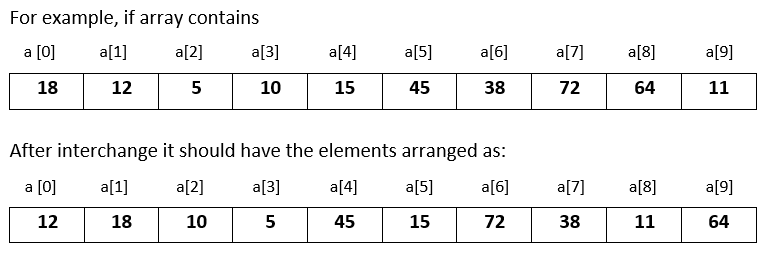# Input 10 numbers in 1d array and interchange the consecutive numbers in Java

###### One Dimensional Array - Question 8

In this question, we will see how to input 10 numbers in a one dimensional integer array and interchange the consecutive numbers in it in Java programming. To know more about one dimensional array click on the one dimensional array lesson.

Q8) Write a program in Java to input 10 numbers in a one dimensional integer array and interchange the consecutive numbers in it. That is, interchange a with a, a with a, a with a and so on.#### Program

``````import java.util.Scanner;

public class Q8
{
public static void main(String args[])
{
int a[]=new int, i,t;
Scanner sc=new Scanner(System.in);

System.out.println("Enter 10 numbers");
for(i=0; i<10; i++)
{
a[i]=sc.nextInt();
}

//Interchanging the consecutive numbers in array
for(i=0; i<10; i=i+2)
{
t=a[i];
a[i]=a[i+1];
a[i+1]=t;
}

System.out.println("\nModified array after interchanging the consecutive numbers");
for(i=0; i<10; i++)
{
System.out.print(a[i]+" ");
}
}
}``````

#### Output

```Enter 10 numbers
18
12
5
10
15
45
38
72
64
11

Modified array after interchanging the consecutive numbers
12 18 10 5 45 15 72 38 11 64```